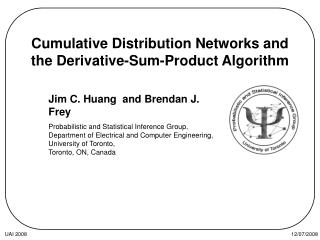# Cumulative Distribution Networks and the Derivative-Sum-Product Algorithm - PowerPoint PPT PresentationDownload PresentationCumulative Distribution Networks and the Derivative-Sum-Product Algorithm

Cumulative Distribution Networks and the Derivative-Sum-Product AlgorithmDownload Presentation## Cumulative Distribution Networks and the Derivative-Sum-Product Algorithm

- - - - - - - - - - - - - - - - - - - - - - - - - - - E N D - - - - - - - - - - - - - - - - - - - - - - - - - - -
##### Presentation Transcript

1. Cumulative Distribution Networks and the Derivative-Sum-Product Algorithm Jim C. Huang and Brendan J. Frey Probabilistic and Statistical Inference Group, Department of Electrical and Computer Engineering, University of Toronto, Toronto, ON, Canada UAI 2008

2. Motivation e.g.: Predicting game outcomes in Halo 2 • Problems where density models may be intractable • e.g.: Modelling arbitrary dependencies • e.g.: Modelling stochastic orderings • Cumulative distribution network (CDN) UAI 2008

3. Cumulative distribution networks (CDNs) • Graphical model of the cumulative distribution function (CDF) • Example: UAI 2008

4. Cumulative distribution functions Negative convergence • Marginalization  maximization • Conditioning  differentiation Positive convergence Monotonicity UAI 2008

5. Necessary/sufficient conditions on CDN functions • Negative convergence (necessity and sufficiency): • Positive convergence (sufficiency): For each Xk, at least one neighboring function  0 All functions  1 UAI 2008

6. Necessary/sufficient conditions on CDN functions • Monotonicity lemma (sufficiency): All functions monotonically non-decreasing… Sufficient condition for a valid joint CDF: Each CDN function can be a CDF of its arguments UAI 2008

7. Marginal independence • Marginalization  maximization • e.g.: X is marginally independent of Y UAI 2008

8. Conditional independence • Conditioning  differentiation • e.g.: X and Y are conditionally dependent given Z • e.g.: X and Y are conditionally independent given Z • Conditional independence  No paths contain observed variables UAI 2008

9. A toy example Required “Bayes net” Markov random fields Check: UAI 2008

10. Inference by message passing • Conditioning  differentiation • Replace sum in sum-product with differentiation • Recursively apply product rule via message-passing with messages , • Derivative-Sum-Product (DSP) … UAI 2008

11. Derivative-sum-product • In a CDN: • In a factor graph: UAI 2008

12. Ranking in multiplayer gaming Player skill functions Player performance Team performance • e.g.: Halo 2 game with 7 players, 3 teams Given game outcomes, update player skills as a function of all player/team performances UAI 2008

13. Ranking in multiplayer gaming = Local cumulative model linking team rank rn with player performances xn e.g.: Team 2 has rank 2 UAI 2008

14. Ranking in multiplayer gaming = Pairwise model of team ranks rn,rn+1 Enforce stochastic orderings between teams via h UAI 2008

15. Ranking in multiplayer gaming • CDN functions = Gaussian CDFs • Skill updates: • Prediction: UAI 2008

16. Results • Previous methods for ranking players: • ELO (Elo, 1978) • TrueSkill (Graepel, Minka and Herbrich, 2006) • After message-passing… UAI 2008

17. Summary • The CDN as a graphical model for CDFs • Unique conditional independence structure • Marginalization  maximization • Global normalization can be enforced locally • Conditioning  differentiation • Efficient inference with Derivative-Sum-Product • Application to Halo 2 Beta Dataset UAI 2008

18. Discussion • Need to be careful when applying to ordinal discrete variables… • Principled method for learning CDNs • Variational principle? (loopy DSP seems to work well) • Future applications to • Hypothesis testing • Document retrieval • Collaborative filtering • Biological sequence search • … UAI 2008

19. Thanks • Questions? UAI 2008

20. Interpretation of skill updates • For any given player let denote the outcomes of games he/she has played previously • Then the skill function corresponds to UAI 2008